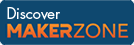Cody

# Problem 44352. The Top 5 Primes

Created by Peng Liu in Community

This problem series invites you to solve two simple problems related to the integer NUMBER FIVE, in order to celebrate Cody's 5th birthday.

In this problem, let's find the top 5 greatest prime numbers along the first non-singleton dimension of an input array.

Example

• If the input is a vector, the output is a length-5 vector containing the top 5 prime numbers of the input sorted in descending order. Append NaNs as needed in the output if the number of primes is less than 5.
```x = 1:10;
y = [7 5 3 2 NaN];
```
• If the input is a m-by-n (m >= 5) matrix, the output is a 5-by-n matrix containing the column-wise top 5 prime numbers of the input matrix sorted in descending order. Whenever there are less than 5 primes found in a specific column of the input, simply append NaNs as needed in the same column of the output.
```% Input x is a matrix
x = [17     6     3
13     8    17
1     2     5
5     3     7
7    11     2
31     7     6];
```
```% Output y
y = [31    11    17
17     7     7
13     3     5
7     2     3
5   NaN     2];
```

Previous problem in this series: Spot the First Occurrence of 5

### Solution Stats

34.99% Correct | 65.01% Incorrect
Last solution submitted on Mar 21, 2019

#### TagsMATLAB and Simulink resources for Arduino, LEGO, and Raspberry Pi